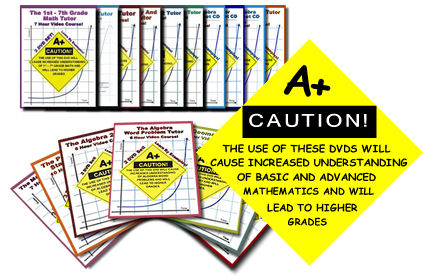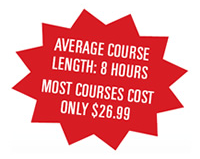# Math Videos on DVD

## "It's like having a tutor right next to you — he explains everything in clear detail."

### Very popular math videos

Do you find math confusing? Need some clear and easy to understand explanations?

Jason Gibson's math videos are a roaring success and I recommend them to you. Go to MathTutorDVD to see what's on offer. Watch some free samples so you get an idea what they are like.

Gibson has a strong academic background but his explanations are easy to follow. The lessons are straightforward, with every step explained in great detail.

The videos are deliberately simple — no bells and whistles here. Gibson stands in front of a basic whiteboard in t-shirt and jeans. This guy’s no stuffy math professor.

### Well-qualified Instructor

Gibson has a Bachelor of Science in Electrical Engineering from the University of Houston, a Masters of Science in Electrical Engineering, and a Masters of Science in Physics.

He worked for Texas instruments and NASA.

### 2 Ways to enjoy the videos

(1) On DVD - at reasonable prices and with low-cost packages available. Learn more!

(2) Online, at \$19.99 per monh. Watch any of the videos - as often as you like! Get over 250 hours of video, from basic math to trigonometry, logarithms, calculus and physics! Worksheets and a discussion forum as well. Join here!

### Are the videos any good?

Let's have a look at some of the math videos on offer.

### Sample Video: The Algebra Word Problem Tutor

Do you panic when you see math questions like the following?

Four times a number increased by two is equal to thirty. What is the number?

In the first video from this 2-DVD set, Introduction To Number Problems, Gibson explains in simple terms how to approach this problem, turn it into algebra and then solve it. The style of the video is friendly and chatty.

Here’s an excerpt from the video:

[Note: The video and audio quality of the DVD that you get is much better than this YouTube sample.]

The full Algebra Word Problem Tutor DVD is 6 Hours in duration. It's a 2-DVD set and contains:

#### Disc 1

• Section 1: Number Problems
• Section 2: Digit Problems
• Section 3: Problems Involving Averages
• Section 4: Coin and Money Problems
• Section 5: Age Problems
• Section 6: Problems Involving Speed, Distance, and Time

#### Disc 2

• Section 7: Mixture Problems
• Section 8: Interest Problems
• Section 9: Lever Problems
• Section 10: Work Problems
• Section 11: Problems Involving Quadratic Equations
• Section 12: Problems Involving Geometry

Get it now! Algebra Word Problem Tutor DVD

### The Full Range of Algebra Videos

Save with Bundled Sets: You can get all these in a bundled set (16 DVD disks - save 8%). Includes 1st to 7th Grade Math Tutor DVDs, Geometry Tutor DVDs and 2 Worksheet CDs.

### Sample Video: Calculus

Here's a sample from one of the calculus videos, What is a Derivative?

[Note: The video and audio quality of the DVD that you get is much better than this YouTube sample.]

The range of topics in Calculus Help: The Calculus 1 & 2 Tutor includes:

#### Disk 1

• Section 1: What Is A Derivative?
• Section 2: The Derivative Defined As A Limit
• Section 3: Differentiation Formulas
• Section 4: Derivatives Of Trigonometric Functions
• Section 5: The Chain Rule
• Section 6: Higher Order Derivatives
• Section 7: Related Rates
• Section 8: Curve Sketching Using Derivatives

#### Disk 2

• Section 9: Introduction To Integrals
• Section 10: Solving Integrals
• Section 11: Integration By Substitution
• Section 12: Calculating Volume With Integrals
• Section 13: Derivatives and Integrals Of Exponentials
• Section 14: Derivatives Of Logarithms
• Section 15: Integration By Parts
• Section 16: Integration By Trig Substitution
• Section 17: Improper Integrals

Order now: Calculus Help: The Calculus 1 & 2 Tutor (2 DVD Set, 8 Hour Course)

### Testimonials

There are many positive testimonials about these videos from satisfied customers. For example:

You have a serious teaching gift.

Proof is, I’m watching your DVDs when I would normally be out and about. Never thought I could learn math. I’m blasting straight through to calculus and then physics. I really enjoy this, and am thinking about a career change. Great job!!!

D. Smith

I panicked as it’s been over 10 years since I last studied math and over 20 years since I’ve looked at any physics book. I was extremely lucky to come across MathTutor DVDs, which in very short time allowed me to come up to speed in 3 areas: trigonometry, physics, and calculus.

S. Deeds-Rubin, mature student, Los Angeles

This comment on the MathTutorDvDs is from Amazon.com

These DVD’s are simple, direct and to the point. They are refreshingly useful and have helped me to learn math concepts I had hurried over in the past or had forgotten. This isn’t bells and whistles, it is the fundamental nuts and bolts of the math that YOU NEED to solve problems from other textbooks.

I ordered the DVD for the library and now recommend it for others whenever I am asked for a good math resource.

JaredMonk, public librarian

There are some very helpful videos here, much cheaper than having your own private tutor.### Large Range of Topics Covered in the Math Videos

The videos cover a large range of topics from pre-algebra to advanced calculus. There are even some physics lessons available as well. Some of the math topics covered (in separate DVDs) include:

• Basic Math
• Word Problems
• Pre-Algebra
• Algebra
• Matrix Algebra
• Geometry
• Trigonometry
• Pre-Calculus
• Calculus
• Unit Conversions
• Probability and Statistics
• Physics (Newtonian Mechanics, Thermodynamics)

### Very Reasonable Cost### AwardsThe MathTutorDVD videos come at a very fair price. Most courses cost less than \$30 (that's for 6 to 8 hours of instruction - less than \$5 per hour) and there are bundles available that bring the cost down even more. You can't get a live tutor for that kind of money!

You can see a lot more sample videos than the ones mentioned above at MathTutorDVD.com (on the left, you'll see "Free Sample Videos").

#### 2 Ways to Access the math videos

(1) On DVD - at reasonable prices and with low-cost packages available. Learn more!

(2) Online, at \$19.99 per month. Watch any of the videos - as often as you like! Get over 250 hours of math videos! Join here!

#### 100% money back guarantee

Every DVD on MathTutorDVD.com comes with a Money Back Guarantee!  If you are not fully satisfied, simply send the DVD back for a full refund.

#### LIMITED TIME BONUS!

Each new MathTutorDVD customer receives 60 minutes of FREE live online tutoring available 24/7 through Tutor.com! A \$35 Value!

Head on over to MathTutorDVD.com right now!